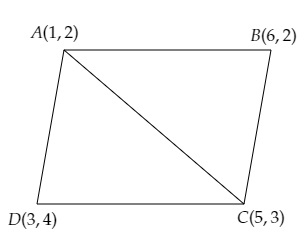# Find the area of the quadrilaterals, the coordinates of whose vertices are$(1, 2), (6, 2), (5, 3)$ and $(3, 4)$

Given:

Vertices of a quadrilateral are $(1, 2), (6, 2), (5, 3)$ and $(3, 4)$.

To do:

We have to find the area of the quadrilateral.

Solution:

Let $A (1, 2), B (6, 2), C(5,3)$ and $D (3, 4)$ be the vertices of a quadrilateral $ABCD$.

Join $A$ and $C$ to get two triangles $ABC$ and $ADC$.This implies,

Area of quadrilateral $ABCD=$ Area of triangle $ABC+$ Area of triangle $ADC$.

We know that,

Area of a triangle with vertices $(x_1,y_1), (x_2,y_2), (x_3,y_3)$ is given by,

Area of $\Delta=\frac{1}{2}[x_{1}(y_{2}-y_{3})+x_{2}(y_{3}-y_{1})+x_{3}(y_{1}-y_{2})]$

Therefore,

Area of triangle $ABC=\frac{1}{2}[1(2-3)+6(3-2)+5(2-2)]$

$=\frac{1}{2}[1(-1)+6(1)+5(0)]$

$=\frac{1}{2}[-1+6]$

$=\frac{1}{2} \times 5$

$=\frac{5}{2}$ sq. units.

Area of triangle $ADC=\frac{1}{2}[1(3-4)+5(4-2)+3(2-3)]$

$=\frac{1}{2}[1(-1)+5(2)+3(-1)]$

$=\frac{1}{2}[-1+10-3]$

$=\frac{1}{2} \times 6$

$=3$ sq. units.

Therefore,

The area of the quadrilateral $ABCD=\frac{5}{2}+3=\frac{5+3(2)}{2}=\frac{11}{2}$ sq. units.

The area of the given quadrilateral is $\frac{11}{2}$ sq. units.

Updated on: 10-Oct-2022

26 Views